# Compensation of Fiber Losses

The transmission distance of any fiber-optic communication system is eventually limited by fiber losses. Until 1995, this loss limitation was mostly overcome using optoelectronic repeaters, devices in which the optical signal is first converted into an electric form using a receiver and then regenerated using a transmitter. Such regenerators become quite complex and expensive for WDM systems because they require demultiplexing of individual WDM channels. An alternative approach to loss management makes use of optical amplifiers, which amplify the entire WDM signal directly without requiring conversion of each channel to the electric domain. Several kinds of optical amplifiers were developed during the 1980s, and the use of optical amplifiers for lightwave systems became widespread during the 1990s. By 1996, optical amplifiers became a part of the fiber-optic cables laid across the Atlantic and Pacific oceans. This tutorial is devoted to the management of fiber losses in long-haul systems. In the first tutorial we discuss the common technique in which optical amplifiers are used periodically along a fiber link and identify the two schemes known as lumped and distributed amplification schemes.

Fiber losses must be compensated for lightwave systems designed to operate over more than 100 km or so because their cumulative effects eventually make the signal so weak that information cannot be recovered at the receiver. In some cases, the use of two lumped amplifiers, one at the transmitter end and the other at the receiver end, can extend the system range to up to 400 km. Since long-haul and submarine lightwave systems extend over thousands of kilometers, fiber losses must be compensated in such systems using a chain of amplifiers that boosts the signal power periodically back to its original value.

#### 1. Periodic Amplification Scheme

Until 1990, the only loss-management technique available to the system designer consisted of inserting an optoelectronic regenerator (often called a repeater) within the fiber link every 80 km or so. A repeater is nothing but a receiver-transmitter pair. In such a device, the optical bit stream is first converted into the electric domain and then regenerated with the help of an optical transmitter. This technique becomes quite cumbersome and expensive for WDM systems as it requires demultiplexing of individual channels at each repeater. A better solution to the fiber-loss problem is to make use of optical amplifiers because they can amplify multiple WDM channels simultaneously. Figure (a) below shows how amplifiers can be cascaded in a periodic manner to form a chain, and thus enable one to transmit an optical bit stream over distances as long as 10,000 km, while retaining the signal in its original optical form.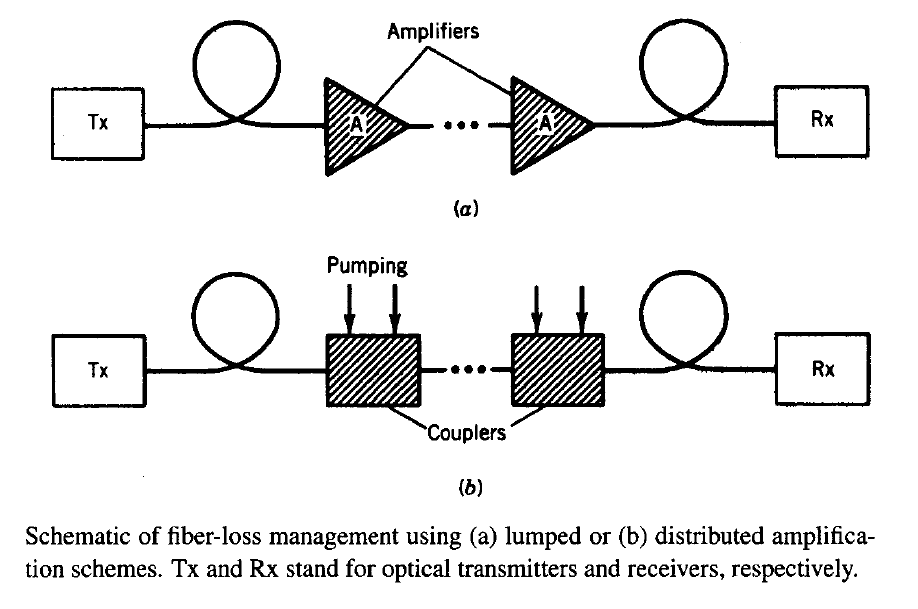Depending on the amplification scheme used, one can divide amplifiers into two categories known as lumped and distributed amplifiers. Most systems employ lumped erbium-doped fiber amplifiers (EDFAs) in which losses accumulated over 60 to 80 km of fiber lengths are compensated using short lengths (~10 m) of erbium-doped fibers. In contrast, the distributed amplification scheme shown in figure (b) above uses the transmission fiber itself for signal amplification by exploiting the nonlinear phenomenon of stimulated Raman scattering (SRS). such amplifiers are known as Raman amplifiers and have been used for lightwave systems since 2002. Their use for loss compensation requires that on e or more pump lasers at suitable wavelengths inject optical power periodically, as shown in figure (b) above.

To understand the periodic amplification scheme, we make use of the following equation that governs propagation of optical signals inside a fiber link.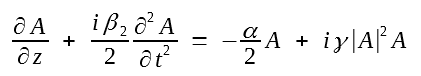The loss parameter α appearing in this equation reduces not only the signal power but it also impacts the strength of the nonlinear effects. This can be seen mathematically by introducing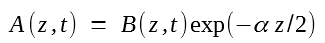and then we can write the first equation in terms of B(z, t) asThe physical interpretation of the preceding second and third equations is clear. The second equation shows that the optical power |A(z, t)|2 decreases exponentially as e-αz at a distance z because of fiber losses. As seen from the third equation, this decrease in the signal power also make the nonlinear effects weaker, as expected intuitively.

The loss in signal power is quantified in terms of the average power defined aswhere we used the A(z, t) equation above and assumed that no other source of energy losses exists so that the integral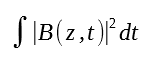over the entire bit stream remains constant in spite of changes in the shape of individual pulses. The average power decreases by a factor of eαL over a fiber of length L. This factor exceeds 20 dB for a 100-km-long fiber cable even in the spectral region near 1.55 μm, where α has the smallest value of around 0.2 dB/km. Numerical values of α depend on the operating wavelength and may exceed 0.4 dB/km in the spectral region near 1.3 μm.

Any loss-management technique based on optical amplifications degrades the signal-to-noise ratio (SNR) of the optical bit stream since all amplifiers add noise to the signal through spontaneous emission. This noise can be included by adding a noise term to the NLS equation together with the gain term. With the addition of such terms, we get the NLS equation as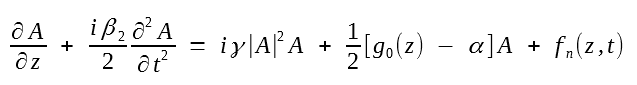where g0(z) is the gain coefficient whose functional form depends on the amplification scheme used. The last term fn(z, t) accounts for the fluctuations induced by spontaneous emission. It vanishes on average, that is, <fn(z, t)> = 0, where angle brackets denote the ensemble average over the random process. If this process is assumed to be Markovian with Gaussian statistics, its statistical properties are completely described by the correlation functionwhere hν0 is the photon energy and the spontaneous emission factor nsp is defined before. The two delta functions in this equation ensure that all spontaneous-emission events are independent of each other both in time and at different points along the amplifier length. We ignore in this section the implications of the noise term in the NLS equation.

#### 2. Lumped versus Distributed Amplification

When EDFAs are used periodically along a fiber link, the length la of each amplifier (typically la < 0.1 km) is much shorter than the spacing LA between two amplifiers. Since g0 = 0 everywhere except within each amplifier, one can solve the standard NLS equation in each fiber section of length LA. Losses in each section reduce the average power by a factor of exp(αLA) and they can be fully compensated by operating each lumped amplifier such that its gain GA = exp(g0la) = exp(αLA). Thus, in a loss-managed long-haul system, EDFAs are inserted periodically after a distance LA and their gain is adjusted such that GA = exp(αLA). It is not necessary that amplifier spacing be uniform throughout the link. In the case of nonuniform spacing, if the nth amplifier is placed at a distance Ln from the transmitter, its gain Gn is chosen to be Gn = exp[α(Ln - Ln-1)] so that each amplifier fully compensates the losses of each fiber span preceding it.

In the case of distributed amplification,should be solved along the entire fiber link, after g0(z) has been determined for a given pumping scheme. It is useful to write the general solution in the form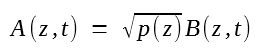where p(z) governs variations in the time-averaged power of the optical bit stream along the link length because of fiber losses and signal amplification. p(z) is found to satisfy a simple ordinary differential equationIf g0(z) were constant and equal to α for all z, the average power of the optical signal would remain constant along the fiber link. This is the ideal situation in which the fiber is effectively lossless. In practice, distributed gain is realized by injecting pump power periodically into the fiber link as shown below.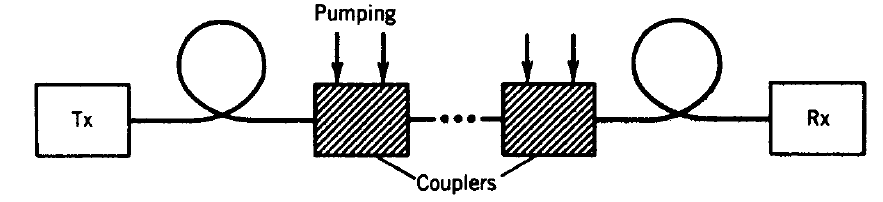Since pump power does not remain constant because of considerable fiber losses at the pump wavelength, g(z) cannot be kept constant along the fiber. However, even though fiber losses cannot be compensated everywhere locally, they can be compensated fully over a distance LA provided the following condition is satisfied:Every distributed amplification scheme is designed to satisfy this equation.  The distance LA is referred to as the pump-station spacing.

As mentioned earlier, stimulated Raman scattering is often used to provide distributed amplification. The scheme works by launching CW power at several wavelengths from a set of high-power semiconductor lasers located at the pump stations. The wavelengths of pump lasers should be int he vicinity of 1.45 μm for amplifying optical signals in the 1.55-μm spectral region. These wavelengths and pump-power levels are chosen to provide a uniform gain over the entire C band (or C and L bands in the case of dense WDM systems). Backward pumping is commonly used for distributed Raman amplification because such a configuration minimizes the transfer of pump-intensity noise to the amplified signal.

#### 3. Bidirectional Pumping Scheme

The use of a bidirectional pumping scheme is beneficial in some cases. To provide physical insight, consider the case in which one pump laser is used at both ends of a fiber section of length LA. In this case, the gain coefficient at a distance z can be written as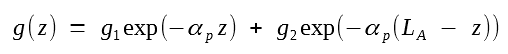where αp is the fiber loss at the pump wavelength and the constants g1 and g2 are related to the pump powers injected at the two ends. Assuming equal pump powers and integratingthe average power of the optical signal, normalized to its fixed value at the pump stations, is found to vary asIn the case of backward pumping, g1 = 0 in the g(z) equation above, and the solution is found to bewhere g2 was again chosen to ensure that p(LA) = 1.

The solid line in the figure below shows how p(z) varies along the fiber in the case of backward pumping for  LA = 50 km using α = 0.2 dB/km and αp = 0.25 dB/km. The case of bidirectional pumping is shown with a dashed line. The case of lumped amplification is also shown for comparison by a dotted line.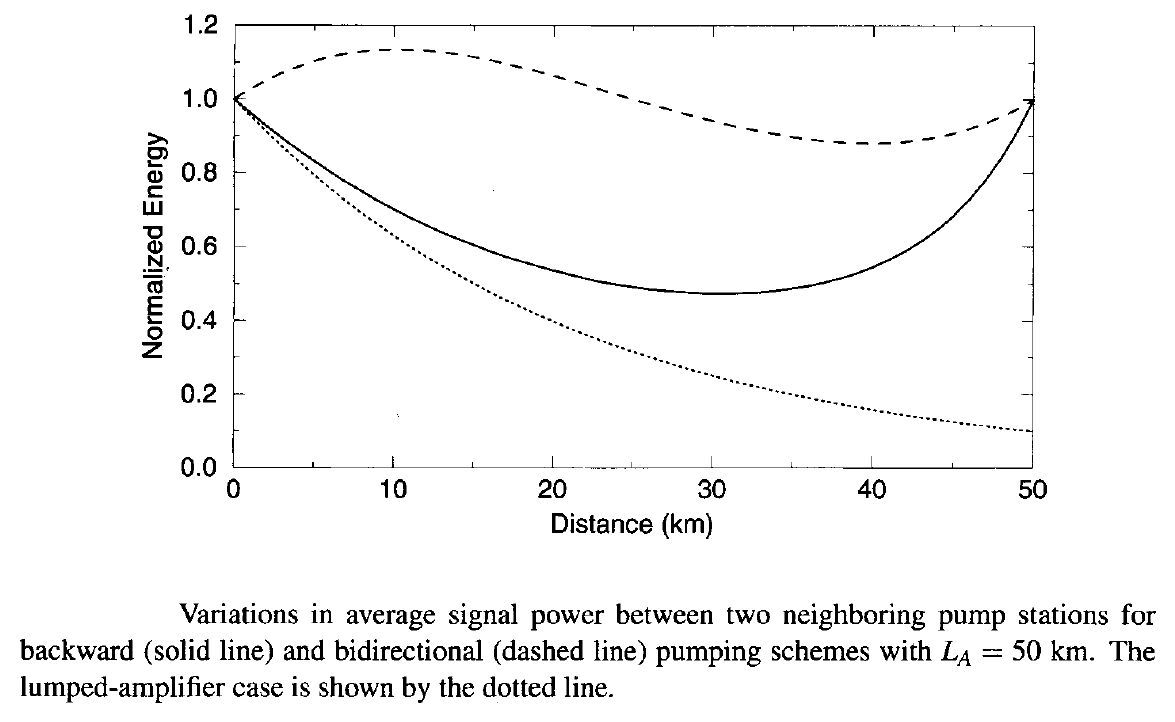Whereas average signal power varies by a factor of 10 in the lumped case, it varies by less than a factor of 2 in the case of backward-pumped distributed amplification. Moreover, it varies by less than 15% in the case of a bidirectional pumping scheme, showing that this scheme is close to the ideal situation in which fiber losses are compensated fully all along the fiber. The range over which p(z) varies depends on the pump-station spacing LA. For example, p(z) varies by a factor of 100 or more when LA = 100 km if lumped amplification is used but by less than a factor of 2 when a bidirectional pumping scheme is employed.

Optical amplifiers developed so far include semiconductor optical amplifiers, doped-fiber amplifiers, Raman amplifiers, and parametric amplifiers. Among these, semiconductor optical amplifiers are rarely used as in-line amplifiers because of issues related to insertion losses, polarization sensitivity, and nonlinear effects such as cross-gain saturation and interchannel crosstalk. Although they are attracting renewed attention in recent years for applications in coarse WDM systems, semiconductor optical amplifiers are not discussed further in this tutorial. Parametric amplifiers, making use of four-wave mixing inside an optical fiber, have attracted considerable attention in recent years. These are also not discussed here as they are still far from being deployed in actual lightwave systems. In contrast, doped-fiber amplifiers are used routinely and Raman amplifiers have also been deployed in some WDM systems. These two types of amplifiers are discussed in detail in the following two tutorials.